# Gradient Descent: One of Machine Learning’s Most Popular Algorithms

Gradient descent is the most popular optimization strategy used in machine learning and deep learning. It is used to train machine learning models and it can also be combined with every algorithms. In addition, it is very easy to understand and implement.

Let’s understand Gradient Descent in detail:

# What is Gradient Descent?

Gradient descent is an optimization algorithm which is used to train a machine learning model. It is an optimization algorithm to find a local minimum of a differential function. It is used to find the values of a function’s coefficients that minimize a cost function as much as possible.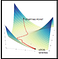Source: Here

It is a first-order iterative optimization algorithm used to minimize the cost function. It is called as first-order optimization algorithm because, in every iteration the algorithm takes the first-order derivative for updating the parameters. The parameters are the coefficients in a regression problem. These parameters are updated by the gradient which gives the direction for the steepest ascent. During each iteration, the parameters are updated in the opposite direction of the gradient computed for cost function with respect to parameters θ. Here the step size is the learning rate α.

To understand gradient descent better, let’s take an example of a man who wants to climb to the top of a hill with the fewest steps along the way as possible. He starts climbing the hill by taking really big steps in the steepest direction until he is not close to the top. Once he reaches close to the top, his steps will get smaller and smaller to avoid overshooting it.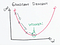Source- Gradient Descent

# How Gradient Descent Works?

It is a better analogy to consider gradient descent as hiking down to the bottom of valley because it is a minimization algorithm which minimizes a given function.

Let’s understand the below equation which describes what gradian descent does: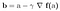Gradient Descent Formula

Here, b is the next position of the mountain climber while a describes his current position. The minus sign is the minimization part of gradient descent. The gamma is the waiting factor and the gradient term Δf(a) is simply the direction of the steepest descent.

In simple words, we can say that this formula derives the next position we need to go which is the direction of the steepest descent.

The following pseudo-code explains the working of the gradient descent:

1. Initialize the parameters with some values. For example, θ1=θ2=…=θn=0
2. By each iteration, keep changing these values in order to minimize the objective function.

# Types of Gradient Descent

There are 3 types of Gradient Descent.

1. Batch Gradient Descent: It uses the complete dataset available to compute the gradient of cost function. Because we need to calculate the gradient on the whole dataset to perform just one update, it can be very slow and is intractable for datasets that don’t fit in memory.
2. Stochastic Gradient Descent (SGD): It takes only a single instance randomly and uses the same in every iteration to compute the gradient of cost function. It uses one training example in every iteration that’s why this algorithm is faster for large dataset. Using SGD, we might not achieve accuracy but the computation results are faster.
3. Mini-batch Gradient Descent: It computes the gradients on small random sets of instances called as mini-batches. It is most favorable and widely used algorithm which makes precise and faster results using a batch of ‘m’ training examples. The common mini-batch sizes range between 50 and 256 but it can be vary for different applications.

Here is the quick comparison of all 3 types of gradient descent algorithm: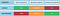Source- Gradient Descent Types

# Summary

In this article, we learned about the basics of gradient descents and its types. It is widely used in machine learning and neural networks. As we have discussed in the article, by choosing the right learning rate we can obtain the local minimum of the cost function. However, finding the right learning rate is hard.

## Definitions:

Local Maximum:

The local maximum is the point where the height of the function at given point is greater than the height anywhere else in the given interval.

Local Minimum:

The local minimum is the point where the height of the function at given point is less than the height anywhere else in the given interval.

It may not be the minimum or maximum for the whole function but locally it is.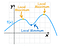Source: Local Maxima and Minima

Learning Rate:

The size of the steps to reach to local minima is called the learning rate. With a high learning rate we can cover more ground each step, but we risk overshooting the lowest point since the slope of the hill is constantly changing. With a very low learning rate, we can confidently move in the direction of the negative gradient since we are recalculating it so frequently. Although the low learning rate is more precise, but calculating gradient is time consuming so it will take long time to get to the bottom.

Cost Function:

The cost function is a measure of how good our model is at making predictions. The shape of a cost function defines our goal of optimization.

If the shape of the cost function is a convex function, our goal is to find the only minimum. This is relatively simpler cause there is no local minimum and we just need to converge to the global minimum.

If the shape of the cost function is not a convex function, our goal is to find the lowest possible value in the neighborhood.

## References:

I am Urmi Parekh and I have been working as a Data engineer for 7+ years. I am pursuing post graduation in AI and DS course at Loyalist college, Toronto.

## More from Urmi Parekh

I am Urmi Parekh and I have been working as a Data engineer for 7+ years. I am pursuing post graduation in AI and DS course at Loyalist college, Toronto.